Additive Subtractive Modeling Solid Modeling Solid modeling is

• Slides: 13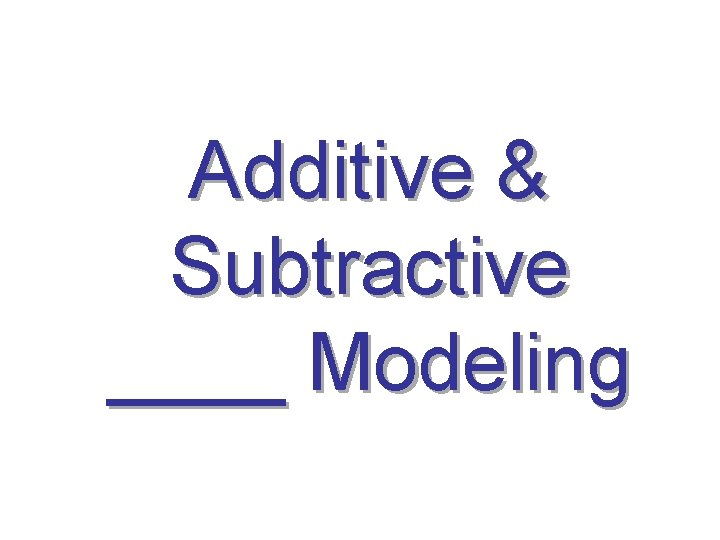Solid Modeling Solid modeling is a type of __ CAD process that represents the _____ of an object, not just its ______ and _____. Wireframe Model Solid Model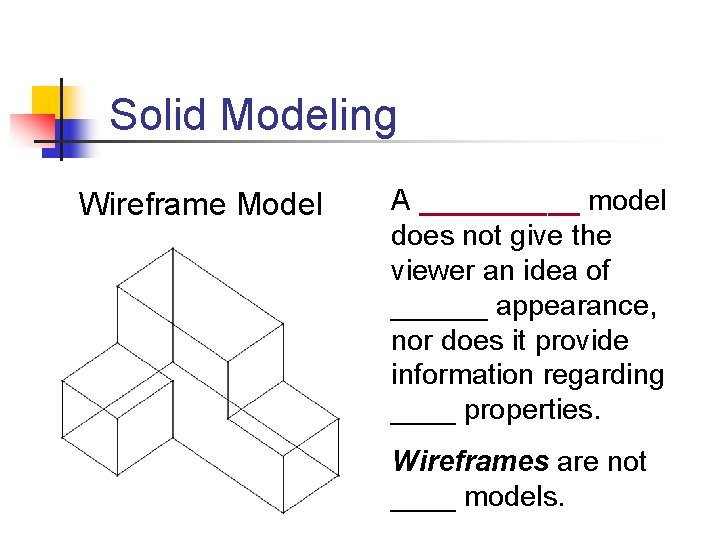Solid Modeling Wireframe Model A _____ model does not give the viewer an idea of ______ appearance, nor does it provide information regarding ____ properties. Wireframes are not ____ models.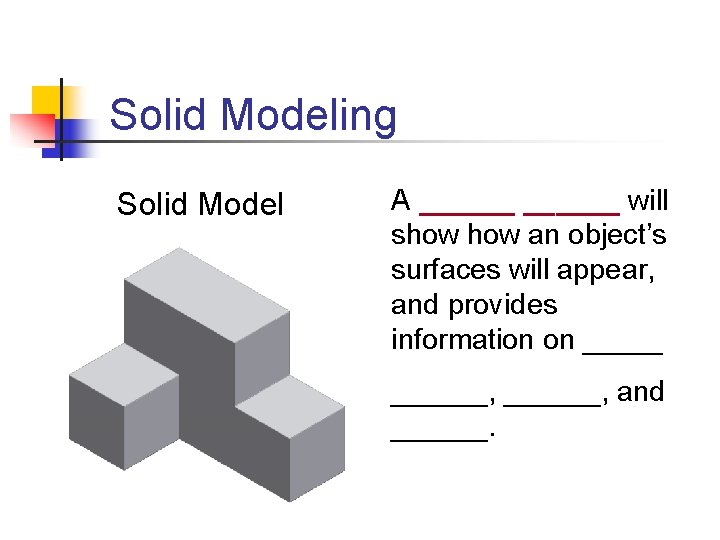Solid Modeling Solid Model A ______ will show an object’s surfaces will appear, and provides information on ______, and ______.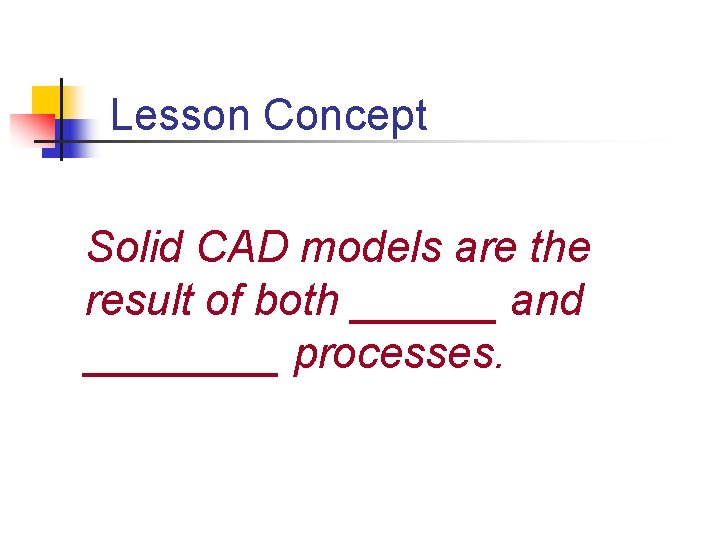Lesson Concept Solid CAD models are the result of both ______ and ____ processes.Solid Modeling Methods All CAD solid modeling programs utilize additive and subtractive modeling methods to create virtual ___ objects. They are also referred to as ______, named after the 18 th century English mathematician Charles Boole.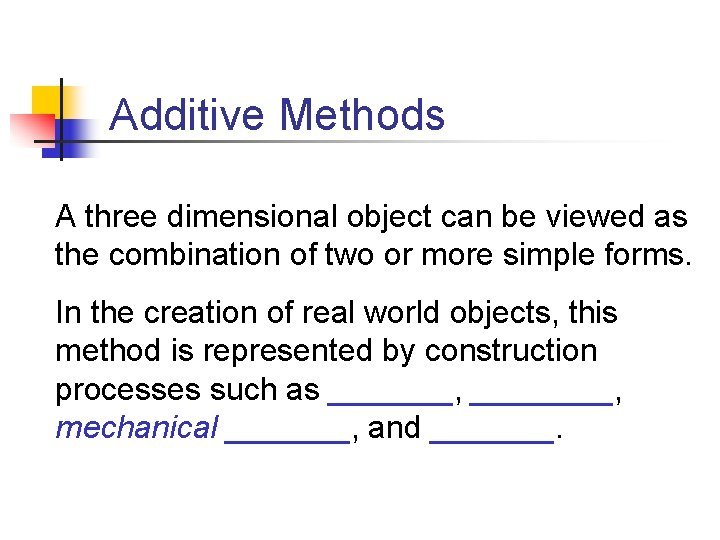Additive Methods A three dimensional object can be viewed as the combination of two or more simple forms. In the creation of real world objects, this method is represented by construction processes such as _______, mechanical _______, and _______.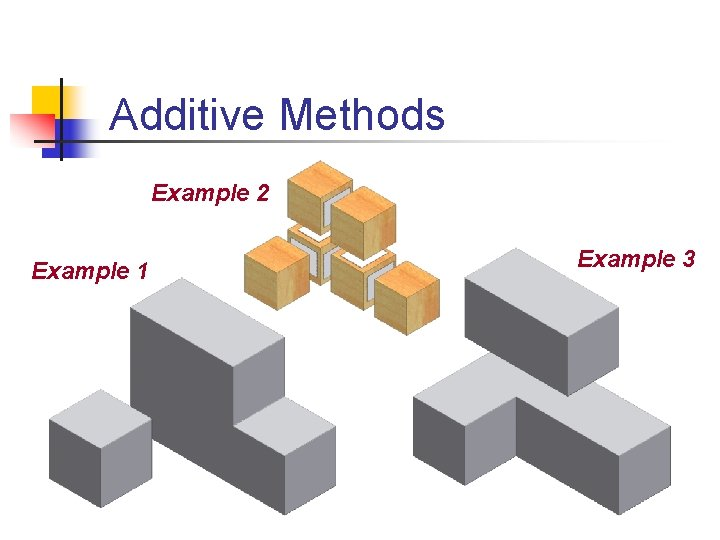Additive Methods Example 2 Example 1 Example 3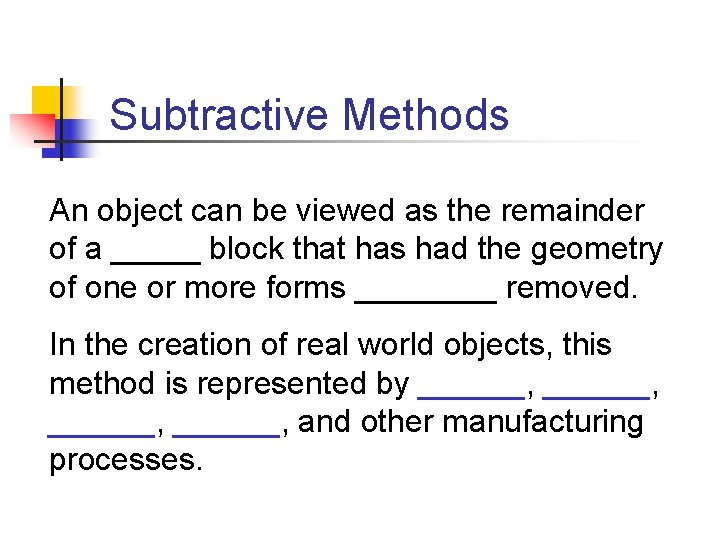Subtractive Methods An object can be viewed as the remainder of a _____ block that has had the geometry of one or more forms ____ removed. In the creation of real world objects, this method is represented by ______, and other manufacturing processes.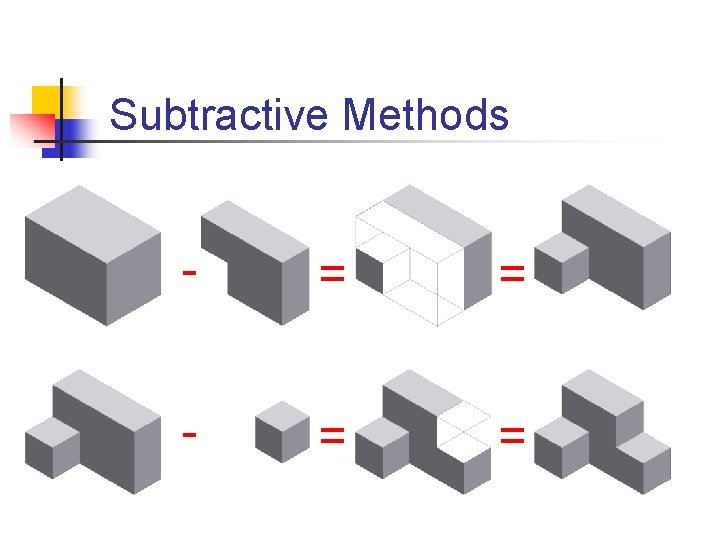Subtractive Methods - = =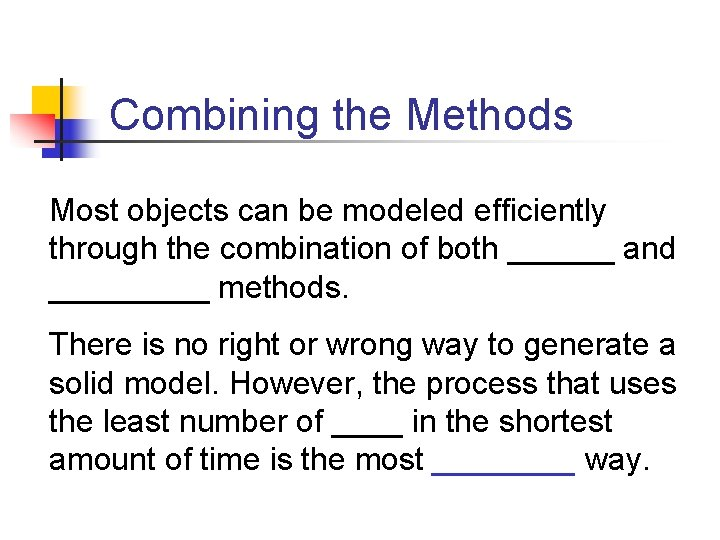Combining the Methods Most objects can be modeled efficiently through the combination of both ______ and _____ methods. There is no right or wrong way to generate a solid model. However, the process that uses the least number of ____ in the shortest amount of time is the most ____ way.Combining the Methods Additive 1. 2. 4. Subtractive 3. Result